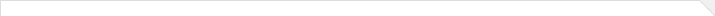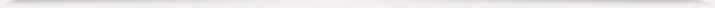报告题目： Soliton resolution for the sine-Gordon equation 报 告 人： 陆冰滢 博士 报告人所在单位： University of Bremen 报告日期： 2021-08-18 星期三 报告时间： 14:30--15:30 报告地点： 腾讯会议 ID: 525 721 484 报告摘要： In this talk, we study the long-time dynamics and stability properties of the sine-Gordon equation f_{tt}−f_{xx}+/sin f=0. Firstly, we use the nonlinear steepest descent for Riemann-Hilbert problems to compute the long-time asymptotics of the solutions to the sine-Gordon equation whose initial condition belongs to some weighted Sobolev spaces. Secondly, we study the asymptotic stability of the sine-Gordon equation. It is known that the obstruction to the asymptotic stability of the sine-Gordon equation in the energy space is the existence of small breathers which is also closely related to the emergence of wobbling kinks. Combining the long-time asymptotics and a refined approximation argument, we analyze the asymptotic stability properties of the sine-Gordon equation in weighted energy spaces. Our stability analysis gives a criterion for the weight which is sharp up to the endpoint so that the asymptotic stability holds. 本年度学院报告总序号： 214